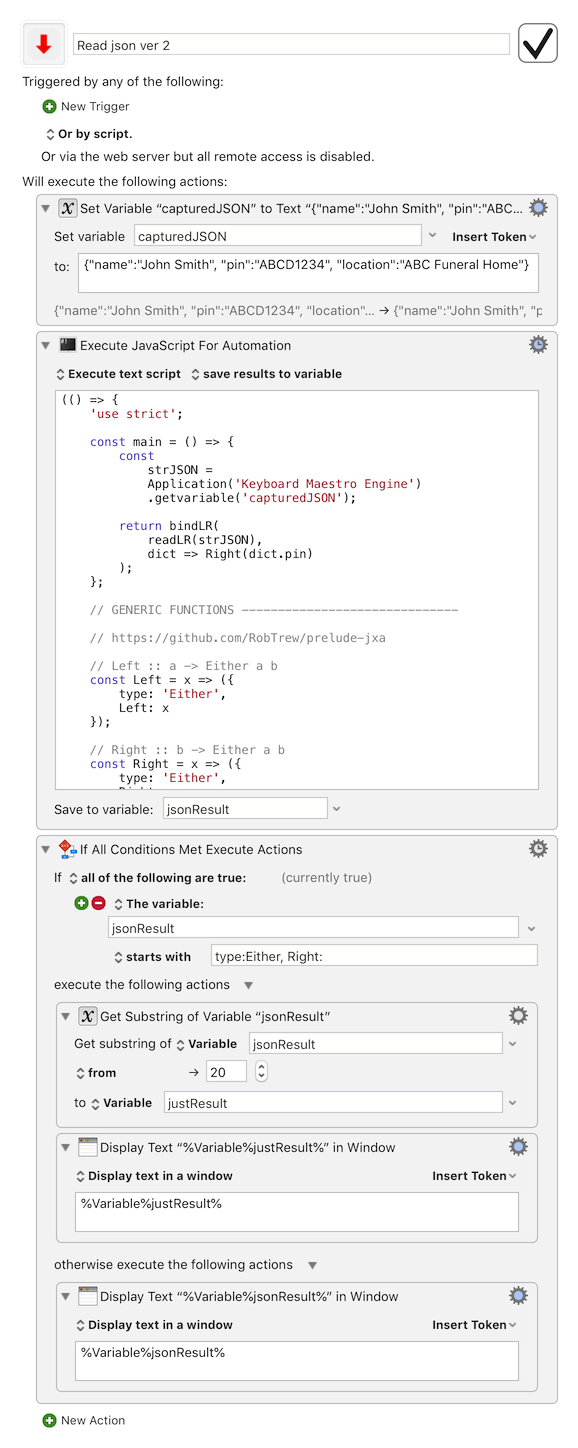# Convert JSON String Variable to JSON object using JXA?

An IT associate has provided a test page to gather variables from an intranet Chrome page. The disabled Action is working fine. I've added a "Set Variable" to the attached macro for testing purposes.

The JXA to convert the JSONstring to a JSONobject and parse into variables is not working. "currentPIN" variables is created but is blank.

Is something wrong with the JXA script? I'd appreciate your expert advice.

``````(function (myJSONstring) {
'use strict';

//parse the string into a useable object
var myJSONobject = JSON.parse(myJSONstring);

//this will return  ABCD1234  and become the value for your KM variable
return myJSONobject.pin;

})(Application('Keyboard Maestro Engine')
.getVariable('capturedJSON'));
``````

The "capturedJSON" string Variable is:
`{name:"John Smith", pin:"ABCD1234", location:"ABC Funeral Home"}`

away from my desk - can’t test but perhaps getVariable is all lower case- that upper V looks unfamiliar to me

(its consistent with the usual JS pattern, of course, but in that library I think it’s setvariable and getvariable - both all lower)

Thanks, ComplexPoint. Same result, unfortunately.

I look again when I get back

Got it. Just two small issues.

• on the JXA side, the only issue is making sure that `.getvariable` is all lower-case.
• on the JSON side, you are just hitting the difference between the relative flexibility of JS code and the particular requirements of standard parseable JSON. In short, object keys don't need quotes (single or double) in JS code, but the JSON standard does require quotes, and specifically double quotes around object keys.

i.e. apart from editing `getVariable` to `getvariable`you just need to ensure that the upstream process is producing conformant JSON.

So this should work:

1 Like

From my notes, I believe the correct function name is:

``````kme.getvariable("capturedJSON")
``````

See Peter's note: Using KM variables in Yosemite Javascript for Applications (JXA)

2 Likes

and if the quality / conformance of the upstream JSON-generation is liable to fluctuate, then it's probably worth trapping any error messages raised by JSON.parse

e.g. sth like:

Read json ver 2.kmmacros (23.2 KB)JS Source

``````(() => {
'use strict';

const main = () => {
const
strJSON =
Application('Keyboard Maestro Engine')
.getvariable('capturedJSON');

return bindLR(
dict => Right(dict.pin)
);
};

// GENERIC FUNCTIONS ------------------------------

// https://github.com/RobTrew/prelude-jxa

// Left :: a -> Either a b
const Left = x => ({
type: 'Either',
Left: x
});

// Right :: b -> Either a b
const Right = x => ({
type: 'Either',
Right: x
});

// bindLR (>>=) :: Either a -> (a -> Either b) -> Either b
const bindLR = (m, mf) =>
undefined !== m.Left ? (
m
) : mf(m.Right);

const readLR = s => {
try {
return Right(JSON.parse(s))
} catch (e) {
return Left(e.message);
};
};

// MAIN ---
return main();
})();
``````

Wow! You all are the greatest. Thanks! I'll pass this along. Getting excited about the benefits of this new approach to capturing variables.

Followup question:
Do I need to create a separate JXA Action to capture each variable defined in the JSON Object or is there a single-script method?

You could create a new KM variable for each Key:Value pair in the dictionary.

(Perhaps adding some kind of prefix to the KM variable names, to distinguish them as a group, and perhaps make it easier to delete them as a group when they are no longer needed)

For example, using Object.entries() to obtain a list of `[key, value]` pairs from the parsed JSON object:

``````(() => {
'use strict';

const main = () => {
const
kme = Application('Keyboard Maestro Engine'),
strJSON = kme.getvariable('capturedJSON');

// Arbitrary prefix to group KM variables of this project
const kmPrefix = 'zzz';

return bindLR(
dict => Right(
unlines(
map(([k, v]) => (
kme.setvariable(kmPrefix + k, {
to: v
}),
kmPrefix + k + '=' + v
),
Object.entries(dict)
)
)
)
);
};

// GENERIC FUNCTIONS ------------------------------

// https://github.com/RobTrew/prelude-jxa

// Left :: a -> Either a b
const Left = x => ({
type: 'Either',
Left: x
});

// Right :: b -> Either a b
const Right = x => ({
type: 'Either',
Right: x
});

// bindLR (>>=) :: Either a -> (a -> Either b) -> Either b
const bindLR = (m, mf) =>
undefined !== m.Left ? (
m
) : mf(m.Right);

// map :: (a -> b) -> [a] -> [b]
const map = (f, xs) => xs.map(f);

// unlines :: [String] -> String
const unlines = xs => xs.join('\n');

const readLR = s => {
try {
return Right(JSON.parse(s))
} catch (e) {
return Left(e.message);
};
};

// MAIN ---
return main();
})();

``````
1 Like

Native support for this is done for the next version:

Note that the JSON will need to be valid, so the field names will need to be properly double-quoted.

3 Likes

Thank you !